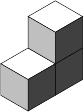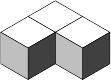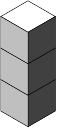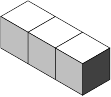Queries for Plane partitions: search statistic / browse statistics / browse maps from / browse maps to

# Definition & Example

• A plane partition $\Lambda = [\lambda^{(1)},\dots,\lambda^{(k)}]$ is a sequence of integer partitions $\lambda^{(i)}$ such that $\lambda^{(i)} \supseteq \lambda^{(i+1)}$ for all $i$.

• Equivalently, it is an array $\Lambda = [\lambda^{(i)}_j]_{ij}$ of positive integers such that $\lambda^{(i)}_j \geq \lambda^{(i+1)}_j$ and $\lambda^{(i)}_j \geq \lambda^{(i)}_{j+1}$ for all well-defined indices $i,j$.

• $\Lambda$ is a plane partition of $n$, denoted by $\Lambda \vdash n$, if $n = n_1 + \dots + n_k$ for $\lambda^{(i)} \vdash n_i$ being a partition of $n_i$.

 the 6 Plane partitions of size 3[,,] [,] [[1,1],] [] [[2,1]] [[1,1,1]]

• Plane partitions are graphically represented by their Ferrers diagram (or Young diagram) as a collection of boxes.

• The number of plane partitions $P(n)$ is A000219. Its generating function is

$$\displaystyle \sum_{n=0}^\infty P(n) x^n = \prod_{k=1}^\infty \frac{1}{(1-x^k)^k}.$$

# Properties

• The number of plane partitions inside an $a\times b\times c$ bounding box is given by MacMahon's box formula:

$$\displaystyle \prod_{i=1}^a \displaystyle \prod_{j=1}^b \displaystyle \prod_{k=1}^c \frac{i+j+k-1}{i+j+k-2}.$$

• There are ten symmetry classes of plane partitions, each of which is enumerated by a nice product formula. These symmetry classes include symmetric, cyclically symmetric, self-complementary, transpose-complementary, and combinations thereof.

# Sage examples

If you want to edit this wiki page, you can download the raw markdown and send your new version to info@findstat.org